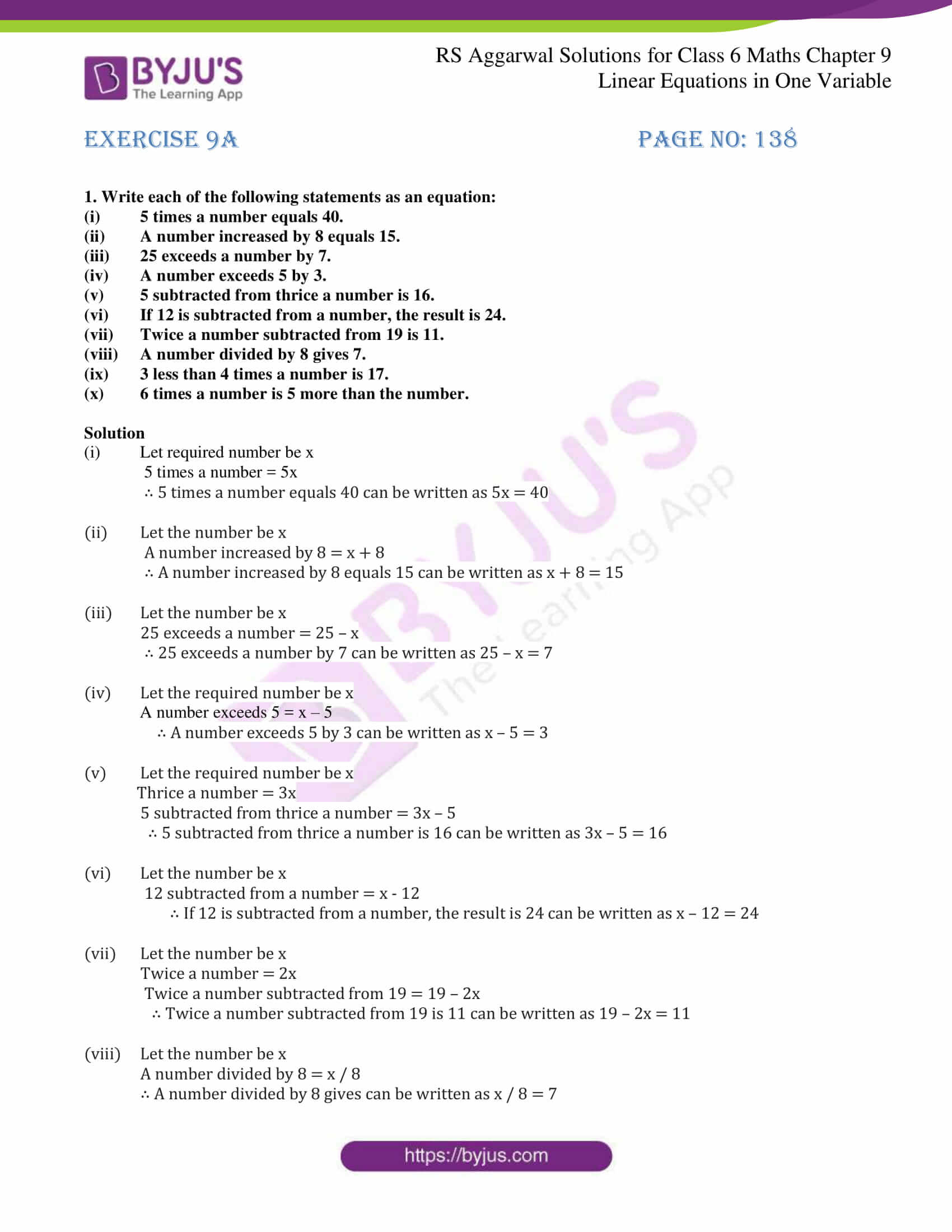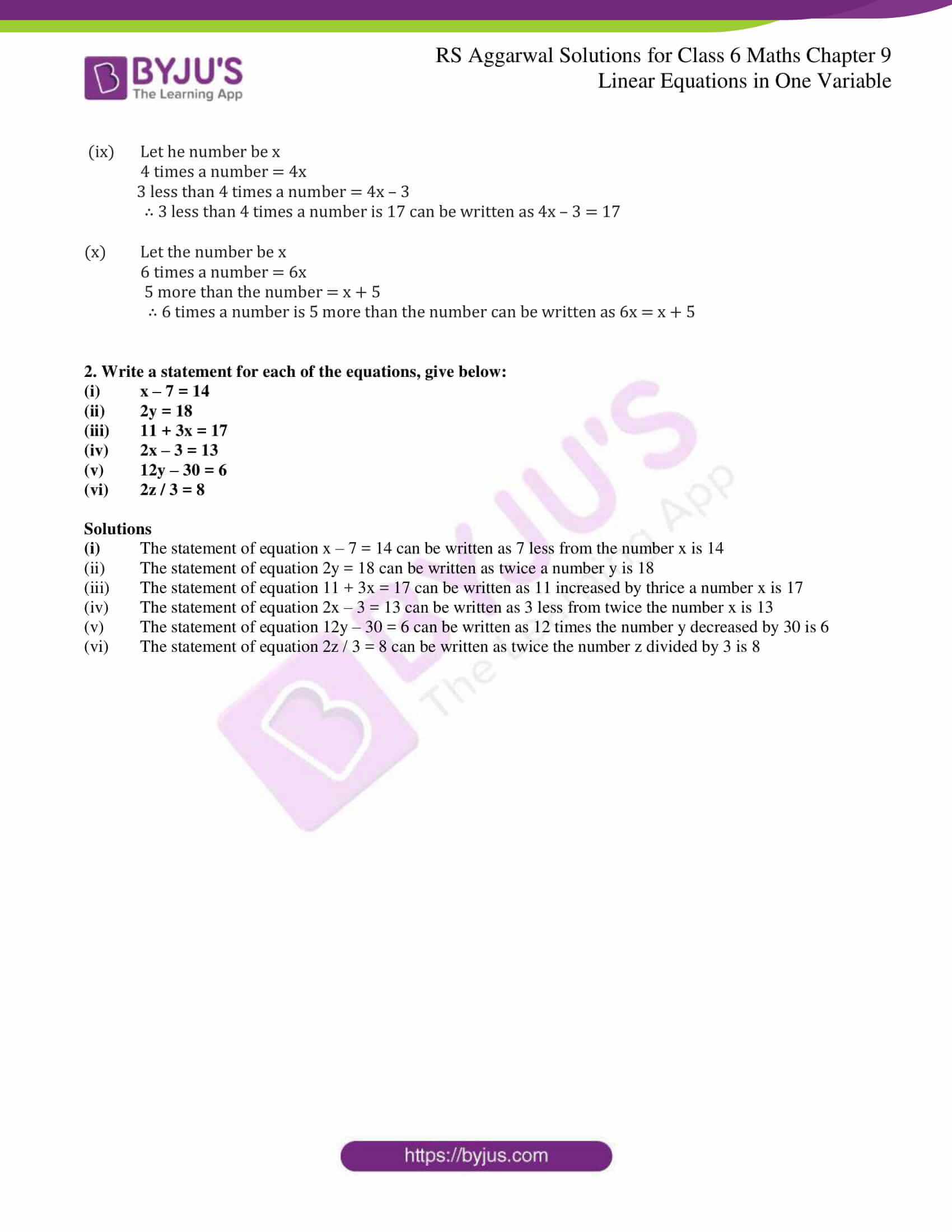# RS Aggarwal Solutions for Class 6 Chapter 9 Linear Equations in One Variable Exercise 9A

RS Aggarwal Solutions for Class 6, Chapter 9 Linear Equations in One Variable, are here for free download by students. An equation in which the highest power of the Variables is 1 is called a Linear Equation. By using the trial and error method, if LHS = RHS for a particular value of the variable, we say that it is the root of the equation. BYJU’S experts have solved RS Aggarwal Solutions for students which are easy to grasp. The accurate answers present in RS Aggarwal Solutions makes learning possible with fun.

## Download PDF of RS Aggarwal Solutions for Class 6 Chapter 9 Linear Equations in One Variable Exercise 9A### Access answers to Maths RS Aggarwal Solutions for Class 6 Chapter 9 Linear Equations in One Variable Exercise 9A

1. Write each of the following statements as an equation:

(i) 5 times a number equals 40.

(ii) A number increased by 8 equals 15.

(iii) 25 exceeds a number by 7.

(iv) A number exceeds 5 by 3.

(v) 5 subtracted from thrice a number is 16.

(vi) If 12 is subtracted from a number, the result is 24.

(vii) Twice a number subtracted from 19 is 11.

(viii) A number divided by 8 gives 7.

(ix) 3 less than 4 times a number is 17.

(x) 6 times a number is 5 more than the number.

Solution

(i) Let required number be x

5 times a number = 5x

∴ 5 times a number equals 40 can be written as 5x = 40

(ii) Let the number be x

A number increased by 8 = x + 8

∴ A number increased by 8 equals 15 can be written as x + 8 = 15

(iii) Let the number be x

25 exceeds a number = 25 – x

∴ 25 exceeds a number by 7 can be written as 25 – x = 7

(iv) Let the required number be x

A number exceeds 5 = x – 5

∴ A number exceeds 5 by 3 can be written as x – 5 = 3

(v) Let the required number be x

Thrice a number = 3x

5 subtracted from thrice a number = 3x – 5

∴ 5 subtracted from thrice a number is 16 can be written as 3x – 5 = 16

(vi) Let the number be x

12 subtracted from a number = x – 12

∴ If 12 is subtracted from a number, the result is 24 can be written as x – 12 = 24

(vii) Let the number be x

Twice a number = 2x

Twice a number subtracted from 19 = 19 – 2x

∴ Twice a number subtracted from 19 is 11 can be written as 19 – 2x = 11

(viii) Let the number be x

A number divided by 8 = x / 8

∴ A number divided by 8 gives can be written as x / 8 = 7

(ix) Let he number be x

4 times a number = 4x

3 less than 4 times a number = 4x – 3

∴ 3 less than 4 times a number is 17 can be written as 4x – 3 = 17

(x) Let the number be x

6 times a number = 6x

5 more than the number = x + 5

∴ 6 times a number is 5 more than the number can be written as 6x = x + 5

2. Write a statement for each of the equations, give below:

(i) x – 7 = 14

(ii) 2y = 18

(iii) 11 + 3x = 17

(iv) 2x – 3 = 13

(v) 12y – 30 = 6

(vi) 2z / 3 = 8

Solutions

(i)The statement of equation x – 7 = 14 can be written as 7 less from the number x is 14

(ii) The statement of equation 2y = 18 can be written as twice a number y is 18

(iii) The statement of equation 11 + 3x = 17 can be written as 11 increased by thrice a number x is 17

(iv) The statement of equation 2x – 3 = 13 can be written as 3 less from twice the number x is 13

(v) The statement of equation 12y – 30 = 6 can be written as 12 times the number y decreased by 30 is 6

(vi) The statement of equation 2z / 3 = 8 can be written as twice the number z divided by 3 is 8